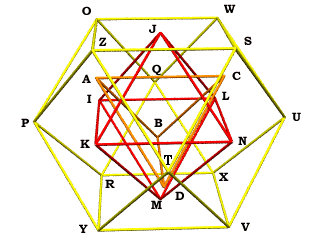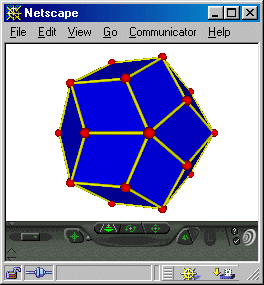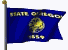# Chapter 7: Playing with Python

by Kirby Urner
First posted: August 28, 1999

In a lot of ways, Python brings us full circle, as many of the features which make it an ideal teaching language were appealing aspects of Xbase (VFP) as well: command line environment, no need to declare variable types, strong objects model. Finally, unlike Microsoft Visual FoxPro, Python is a free language, and cross-platform to boot.

I'm repeating the graphic at right because Python proves very well suited to this "points dictionary" approach to defining various polyhedra as subclasses which share A-Z in common.

In the Java implementation, I used a Hashtable class to store vertices, edges and faces by name. Python makes this even easier by:

1. having a built-in "dictionary" type, with easy syntax for storing (name, object) pairs and
2. incorporating such a dictionary into the definition of every class, to internally collect its variables and methods.Not shown: inverted tetrahedron EFGH
Whereas the Java syntax for adding face definitions was as follows:
```  fdict = new Hashtable(4);
```

In Python I simply write (in my definition of the subclass for a Tetrahedron):

```  faces = (('A','B','C'),('A','B','D'),('A','C','D'),('B','C','D'))
```

where the points A,B,C and D have already been defined as part of the superclass data dictionary.

The points A-Z are defined using of a new Vector class which incorporates another attractive feature in Python, not available in either the current Java or VFP: operator overloading. By writing methods for the predefined function names __mul__, __add__, __neg__ and a few others, we can change the behavior of such primitive operators as *,+, - with regard to objects (e.g. vectors and polyhedra). This means we can perform vector addition very straightfowardly in code, once the function definition for vector addition has been made a part of our Vector objects:

```  #A-Z form the basic "vocabulary" of needed vertices. The Rhtriac
#subclass jitterbugs O-Z to support derivative points a-t (lowercase)
#(Icosa and Pdodeca are both subclasses of Rhtriac)

E=-A; F=-B; G=-C; H=-D

I=A+B; J=A+C; K=A+D; L=B+C; M=B+D; N=C+D
O=I+J; P=I+K; Q=I+L; R=I+M; S=N+J; T=N+K
U=N+L; V=N+M; W=J+L; X=L+M; Y=M+K; Z=K+J
```

Here's our definition of the class method for vector addition, which returns a new vector as output:

```   def __add__(self,pVector):
if type(pVector)==type(self):
outlist = []
for i in range(4): outlist.append(self.data[i]+pVector.data[i])
else: outlist = self.data
```

Once A-Z have been defined in the Poly class -- by setting A-D equal to basis quadrays, and developing the others using negation and vector addition -- we can simply "look them up by name" in the native class dictionary, which is named __dict__. We get this ability "for free", without needing to define any additional dictionaries and/or hashtables.

The interactive dialog below shows the user instantiating a new rhombic dodecahedron and querying the object's built-in dictionary:

```   Python 1.5.2 (#0, Apr 13 1999, 10:51:12) [MSC 32 bit (Intel)] on win32
Copyright 1991-1995 Stichting Mathematisch Centrum, Amsterdam
>>> import polys
>>> oRhdodeca = polys.Rhdodeca()
>>> oRhdodeca.__dict__['F']
Vector(1, 0, 1, 1)
>>> oRhdodeca.__dict__['G']
Vector(1, 1, 0, 1)
>>>
```

As in earlier chapters, I'm using "quadray format" integrally within my class methods. In fact, this application uses quadray 4-tuples as the native format for the Vector object, by which I mean the data variable unique to each vector is a quadray 4-tuple. Tuples are "immutable" in Python, meaning you can't do in-place changes to their members (unlike lists, which are mutable). Using tuples for vector data helps ensure that my vector operations return new vectors, but without affecting the ones operated upon.

 The quadray approach received a boost since I wrote Chapter 6 thanks to Tom Ace, who derived some methods for doing vector cross and dot products that work directly with the 4-tuples, along with some rotation matrices, and a simplified distance formula (thanks Tom!). At right are colored hoops corresponding the the path taken by a basis quadray when rotated around a basis quadray of the same color. For example, vector B (red) rotated around vector A (cyan) goes in a circle through C (blue) and D (green).Given that vectors can be scaled, translated and rotated, our polyhedra, which consist of vectors, do the same things, likewise with operator overloading. oTetra * 3 is a tetrahedron 3 times larger than oTetra. As is the case with vectors, operations on polyhedra return new polyhedra, do not change the existing ones in place. My Python implementation includes optional meshing of triangular and square faces, mesh curvature (pushing vertices an equal distance from a common origin), and VRML output (see Chapter 5 for more about these features). To run the Python code yourself, you'll need an installed version of the Python environment. Note that in recent versions of the source I've removed any dependence on the useful NumPy modules from Lawrence Livermore National Labs. This was to facilitate experiments with JPython, which compiles to a Java Virtual Machine (JVM). Some of NumPy's Python extensions were compiled directly from C and were therefore not reusable in the Java context.Download my code into a subdirectory and include this in your PYTHONPATH. Then test the code by importing ps, which contains a number of test programs. For example: ``` Python 1.5.2 (#0, Apr 13 1999, 10:51:12) [MSC 32 bit (Intel)] on win32 Copyright 1991-1995 Stichting Mathematisch Centrum, Amsterdam >>> import ps >>> ps.testprog6() >>> ps.testprog13() ``` These test programs will generate a colorful ray tracing, and VRML rhombic triacontahedron respectively, both depicted above. To render the ray tracing, output by testprog6() as hoops.pov (a text file), you will need to install POV-ray (freeware, also Linux-compatible). To inspect the output of testprog13(), a file named PyRhtriac.wrl (also a text file), you'll need a VRML viewer -- often a web browser plug-in (CosmoPlayer 2.0 for the Netscape browser is what's shown above). On-line Resources: Chapters I, 2, 3, 4, 5 and 6 of this webtext Python language website More background pages on using Python + Povray "dynamic duo" PyPolys.zip (source code). Last modified: May 30, 2000 Postings about this application to comp.lang.python [ 2] Re: Python and Quadrays (Aug 19, 1999 to geometry-research, Math Forum) Re: Python and Pascal's Triangle (Jan 18, 2000 to geometry-pre-college) NumPy from Lawrence Livermore National Labs Computer Programming for Everybody (Revised Proposal, July 1999) Some debate regarding Python vs Scheme (comp.lang.python, February, 2000) with some follow-up remarks (Feb 2, 2000) Return to Symbols and OperatorsOregon Curriculum Network